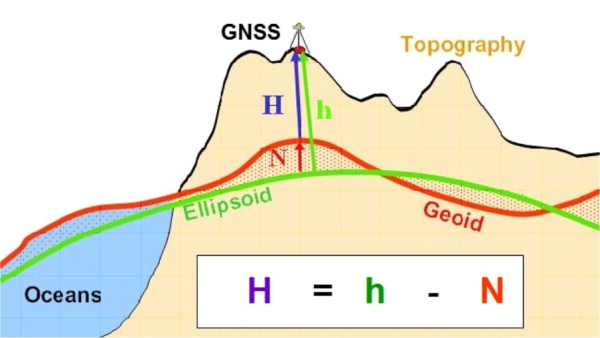### Conversion of Ellipsoidal GNSS Heights h to Physical Heights H by a Height Reference Surface Database N=N(p|B,L,h)

The digital finite-element height reference surface (DFHRS) research and development project (www.dfhbf.de) (German word DHFBF = Digitale Finite Elemente Höhenbezugsfläche) is aiming at the computation of a parametrized and georeferenced height reference surface (HRS), called N=N(p|B,L,h) in the following (fig. 1). The HRS parameters p are stored in a DFHRS data-base (DFHRS_DB).

Depending on the height system type, physical heights H (fig 1) are called the orthometric heights, the normal heights (the European height system), or the spheroidal normal heights (NN-heights). The respective HRS, abbreviated with N (fig. 1), is accordingly either the geoid, the QGeoid or the NN-surface. N is always the height of the HRS above the reference ellipsoid.Fig. 1.: The principle of GNSS-based height determination: H = h  N.

The main practical target of the DFHRS project dealing with the development of integrated approaches for the computation of Geoid or QGeoid HRS N (fig. 1) is to enable the conversion of ellipsoidal GNSS heights h (determined at the earth topography) into the physical earth gravity field based standard, or sea-level heights, H by the direct transformation H = h  N.